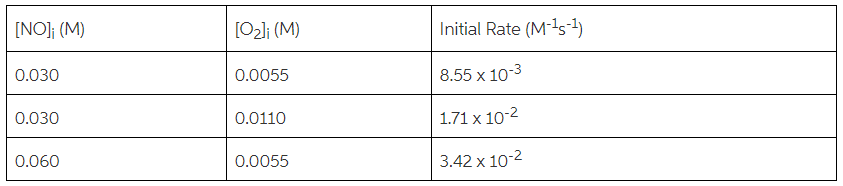# Problem: Determine the rate law and the value of k for the following reaction using the data provided.2 NO(g) + O2(g) → 2NO2(g)

###### FREE Expert Solution
88% (202 ratings)
###### FREE Expert Solution

We’re being asked to calculate the rate law and the value of the rate constant (k) based on the reaction and data given.

Recall that the rate law only focuses on the reactant concentrations and has a general form of:

k = rate constant
A & B = reactants
x & y = reactant orders

We’re going to calculate the rate law and the rate constant using the following steps:

88% (202 ratings)###### Problem Details

Determine the rate law and the value of k for the following reaction using the data provided.

2 NO(g) + O2(g) → 2NO2(g)What scientific concept do you need to know in order to solve this problem?

Our tutors have indicated that to solve this problem you will need to apply the Rate Law concept. You can view video lessons to learn Rate Law. Or if you need more Rate Law practice, you can also practice Rate Law practice problems.

What is the difficulty of this problem?

Our tutors rated the difficulty ofDetermine the rate law and the value of k for the following ...as high difficulty.

How long does this problem take to solve?

Our expert Chemistry tutor, Dasha took 9 minutes and 24 seconds to solve this problem. You can follow their steps in the video explanation above.

What professor is this problem relevant for?

Based on our data, we think this problem is relevant for Professor Lirag's class at University of Houston-Clear Lake.